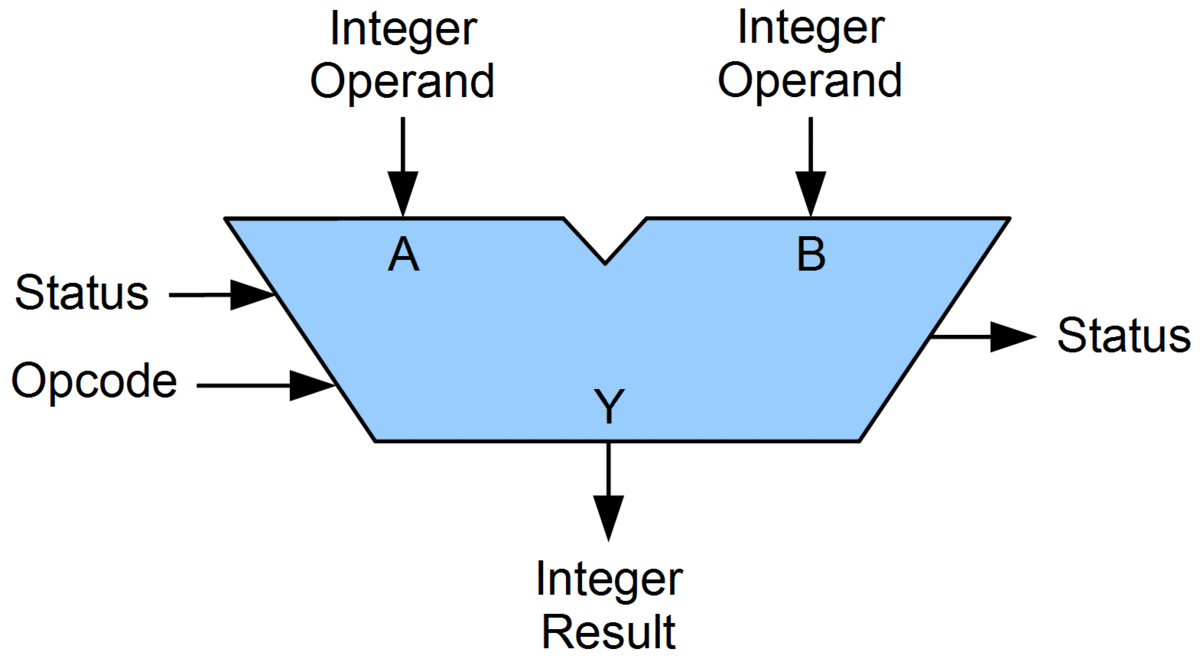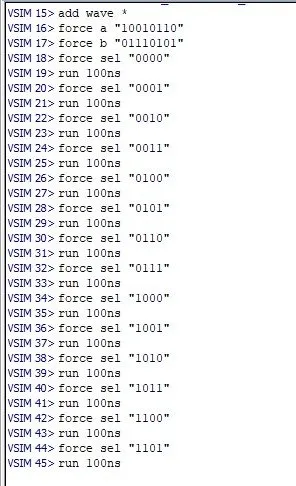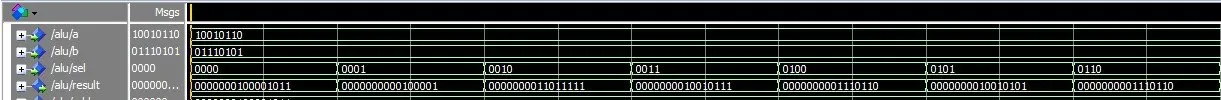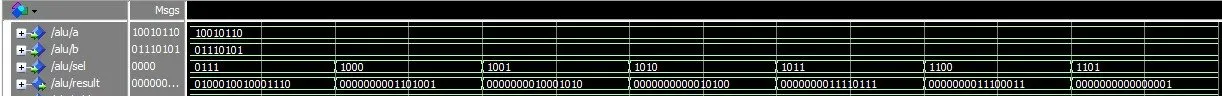# Logic Design - VHDL Simple ALU Circuit (part 3)Hello its me again! Today we will end our Series of creating a Simple ALU Circuit in VHDL with part 3. We will combine all the Components we talked about in part 1 and part 2 in a structural VHDL representation that we will also simulate! So, without further do, let's get started!

# ALU Coding:

Let's start out with the Code. Our ALU will have two 8-bit inputs a and b, a selection input of 4-bit size and a 16-bit result! Because, we will write it in structural VHDL we then have to define all the Components that will be all the ones we wrote until know! The selection line will be put inside of an with select statement and so we will have a signal for each individual operation that will be passed to the result/output depending on the selection line. For the most Component we will need a simple port map. The boolean operations will be done here using a AND, OR or XOR Gate on each bit! The most complicated operation will be the comparison that will need two 4-bit comparators and help signals that check the outputs of the 2 comparators and give the right value to the comp signal, that will be 0 when equal, 1 when a>b and -1 when a</p>

So, our Code looks like this (and yes it is big):

``library ieee;``
``use ieee.std_logic_1164.all;``
``use ieee.numeric_std.all;``
``entity alu is``
``port(``
``    a, b: in std_logic_vector(7 downto 0);``
``    sel: in std_logic_vector(3 downto 0);``
``    result: out std_logic_vector(15 downto 0)``
``);``
``end alu;``

``architecture arch of alu is``

``component adder_8_bit``
``port(``
``    a, b: in std_logic_vector(7 downto 0);``
``    s: out std_logic_vector(7 downto 0);``
``    c_out: out std_logic``
``);``
``end component;``

``component subber_8_bit``
``port(``
``    a, b: in std_logic_vector(7 downto 0);``
``    s: out std_logic_vector(7 downto 0)``
``);``
``end component;``

``component incrementor_numeric``
``port(``
``    src: in std_logic_vector(7 downto 0);``
``    result: out std_logic_vector(15 downto 0)``
``);``
``end component;``

``component decrementor_numeric``
``port(``
``    src: in std_logic_vector(7 downto 0);``
``    result: out std_logic_vector(15 downto 0)``
``);``
``end component;``

``component multiplier_numeric``
``port(``
``    src1, src2: in std_logic_vector(7 downto 0);``
``    result: out std_logic_vector(15 downto 0)``
``);``
``end component;``

``component and2_gate``
``port(``
``    a, b: in std_logic;``
``    c: out std_logic``
``);``
``end component;``

``component or2_gate``
``port(``
``    a, b: in std_logic;``
``    c: out std_logic``
``);``
``end component;``

``component xor2_gate``
``port(``
``    a, b: in std_logic;``
``    c: out std_logic``
``);``
``end component;``

``component digital_comparator_dataflow``
``port(``
``    a: in std_logic_vector(3 downto 0);``
``    b: in std_logic_vector(3 downto 0);``
``    equal: out std_logic;``
``    greater: out std_logic;``
``    less: out std_logic``
``);``
``end component;``

``signal add, sub1, sub2, incr_a, incr_b, decr_a, decr_b, mul, comp, ``
``not_a, not_b, a_and_b, a_or_b, a_xor_b : std_logic_vector(15 downto 0);``
``signal equal, greater, less: std_logic_vector(1 downto 0);``
``signal help1, help2, is_equal, is_greater, is_less: std_logic;``

``begin``

``adder: adder_8_bit port map(a, b, add(7 downto 0), add(8));``
``subber1: subber_8_bit port map(a, b, sub1(7 downto 0));``
``subber2: subber_8_bit port map(b, a, sub2(7 downto 0));``
``incr1: incrementor_numeric port map(a, incr_a);``
``incr2: incrementor_numeric port map(b, incr_b);``
``decr1: decrementor_numeric port map(a, decr_a);``
``decr2: incrementor_numeric port map(b, decr_b);``
``multiplier: multiplier_numeric port map(a, b, mul);``
``and1: and2_gate port map(a(7), b(7), a_and_b(7));``
``and2: and2_gate port map(a(6), b(6), a_and_b(6));``
``and3: and2_gate port map(a(5), b(5), a_and_b(5));``
``and4: and2_gate port map(a(4), b(4), a_and_b(4));``
``and5: and2_gate port map(a(3), b(3), a_and_b(3));``
``and6: and2_gate port map(a(2), b(2), a_and_b(2));``
``and7: and2_gate port map(a(1), b(1), a_and_b(1));``
``and8: and2_gate port map(a(0), b(0), a_and_b(0));``
``or1: or2_gate port map(a(7), b(7), a_or_b(7));``
``or2: or2_gate port map(a(6), b(6), a_or_b(6));``
``or3: or2_gate port map(a(5), b(5), a_or_b(5));``
``or4: or2_gate port map(a(4), b(4), a_or_b(4));``
``or5: or2_gate port map(a(3), b(3), a_or_b(3));``
``or6: or2_gate port map(a(2), b(2), a_or_b(2));``
``or7: or2_gate port map(a(1), b(1), a_or_b(1));``
``or8: or2_gate port map(a(0), b(0), a_or_b(0));``
``xor1: xor2_gate port map(a(7), b(7), a_xor_b(7));``
``xor2: xor2_gate port map(a(6), b(6), a_xor_b(6));``
``xor3: xor2_gate port map(a(5), b(5), a_xor_b(5));``
``xor4: xor2_gate port map(a(4), b(4), a_xor_b(4));``
``xor5: xor2_gate port map(a(3), b(3), a_xor_b(3));``
``xor6: xor2_gate port map(a(2), b(2), a_xor_b(2));``
``xor7: xor2_gate port map(a(1), b(1), a_xor_b(1));``
``xor8: xor2_gate port map(a(0), b(0), a_xor_b(0));``

``add(15 downto 9) <= "0000000";``
``sub1(15 downto 8) <= "00000000";``
``sub2(15 downto 8) <= "00000000";``
``incr_a(15 downto 9) <= "0000000";``
``incr_b(15 downto 9) <= "0000000";``
``decr_a(15 downto 9) <= "0000000";``
``decr_b(15 downto 9) <= "0000000";``
``not_a(15 downto 8) <= "00000000";``
``not_b(15 downto 8) <= "00000000";``
``not_a(7 downto 0) <= not a;``
``not_b(7 downto 0) <= not b;``
``a_and_b(15 downto 8) <= "00000000";``
``a_or_b(15 downto 8) <= "00000000";``
``a_xor_b(15 downto 8) <= "00000000";``

``comp1: digital_comparator_dataflow port map(a(7 downto 4), b(7 downto 4), equal(1), greater(1), less(1));``
``comp2: digital_comparator_dataflow port map(a(3 downto 0), b(3 downto 0), equal(0), greater(0), less(0));``
``and_is_equal: and2_gate port map(equal(1), equal(0), is_equal);``
``and_help1: and2_gate port map(equal(1), greater(0), help1);``
``or_is_greater: or2_gate port map(greater(1), help1, is_greater);``
``and_help2: and2_gate port map(equal(1), less(0), help2);``
``or_is_less: or2_gate port map(less(1), help1, is_less);``
``comp <= "0000000000000000" when is_equal='1' else``
`` "0000000000000001" when is_greater='1' else``
`` "1111111111111111" when is_less='1';``
``     ``
``with sel select ``
``result <= add when "0000",``
``   sub1 when "0001",``
``   sub2 when "0010",``
``   incr_a when "0011",``
``   incr_b when "0100",``
``   decr_a when "0101",``
``   decr_b when "0110",``
``   mul when "0111",``
``   not_a when "1000",``
``   not_b when "1001",``
``   a_and_b when "1010",``
``   a_or_b when "1011",``
``   a_xor_b when "1100",``
``   comp when others;``
``end arch;``

I hope that you don't hate VHDL now, cause yes it really gets tense when writing even such a simple behavior, but think about some things that we could have made in another way. We could for example use a AND, OR and XOR Gate for 8-bits or even have a 8-bit comparator or a 8-bit comparator written using two 4-bit comparators, but just use the 8-bit inside of the ALU! But I think that if you understand what I did here you will now know VHDL even better, cause this Coding is actually not so good and would not be recommended by anyone. That way you can make tweaks and changes by your own and see what works for you!

# ALU Simulation:

To Compile this Circuit we will have to start compiling from the bottom to the top, starting off with the Basic Gates that some Components are build off, compiling all the components again in a order needed and finally compiling and simulating the ALU Circuit!

So, we will start off with the Basic Gates AND2, OR2 and XOR2, then compile the Half Adder, Full Adder and Adder 8-bit and Subber 8-bit Circuits in that Order, but the last two can switch places. After that we compile the comparator, incrementor, decrementor and multiplier that are dataflow and don't need any order and could also be compiled even before the Gates, and finally after compiling every Component we can also compile and simulate the ALU!

Simulation in Modelsim:You can see that we set a specific value for a and b and then simple change the sel input to each possible operation and the result changes! Also, b-a gives us a negative result that we can detect by seeing that the output is greater then both inputs.

I won't get into writing a Testbench, cause its relatively simple and you can try it out by yourself using my assignment inside of Modelsim :P

And this is actually it and I hope you enjoyed it!

This is also the final post of our little Series. I will get into VHDL again in some time, but I don't have something more ready for now and so we will see ;)

Until next time Bye!

H2
H3
H4
3 columns
2 columns
1 column
Join the conversation now